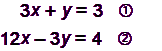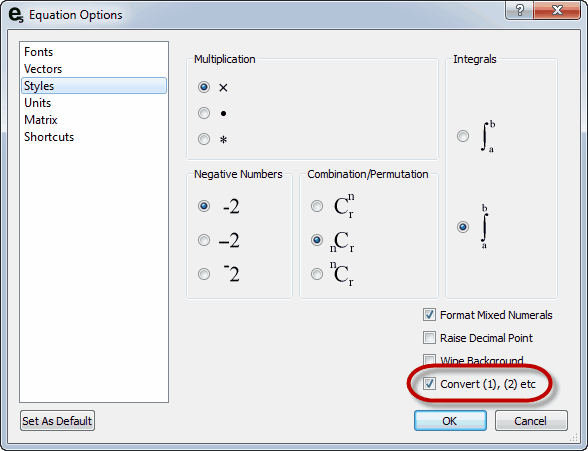﻿ Equation Numbers

# Equation Numbers

Top  Previous  Next
 FX Equation can add equation numbers to equations.  These are useful when defining systems of simultaneous equations.   Equation numbers are entered by surrounding a digit with parentheses.   Example:is entered as   3x+y=3   (1) 12x-3y=4   (2)   FX Equation will only render equation numbers when the equation number has some white space around it.  For example, if you type sin(3), FX Equation will NOT convert the (3) to an equation number.   You must have the following option checked to use equation numbers.